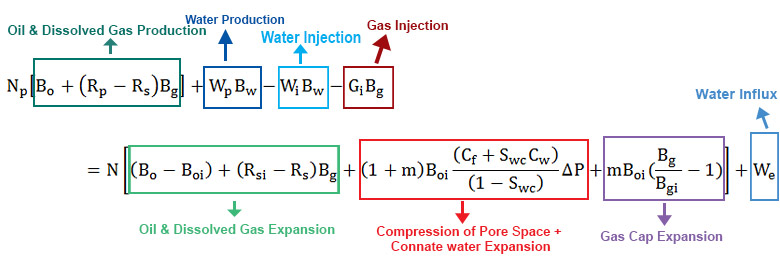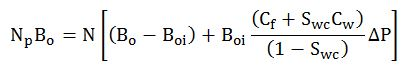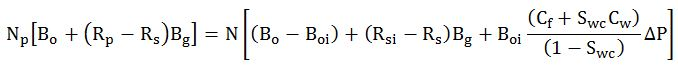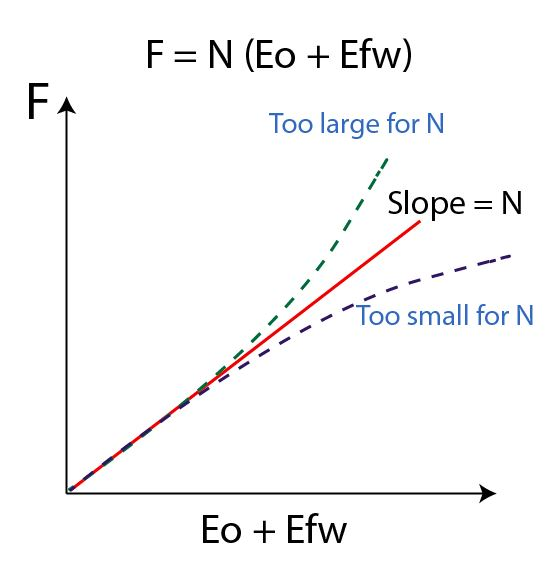# Solution Gas Drive Mechanism Explained in Material Balance Equation

Material balance equation can be applied for any drive mechanism and this article demonstrates how to apply the material balance equation in a solution drive mechanism. For a solution drive mechanism, there are 2 cases. The first case is when reservoir pressure is above a bubble point and the second case is when reservoir pressure is below a bubble point.

Solution Gas above Bubble PointFigure 1 – Full Material Balance Equation

Assumptions

• No water production
• No water injection
• No gas injection
• No gas cap
• No water influx

Fact

Rs = Rsi

Cancel out parameters to match with solution gas assumptions.Figure 2 – Cancel out some parameters

The material balance will be like this:Oil Compressibility is the equation below;

Co = (Bo – Boi) ÷ (Boi ×ΔP)Solution Gas below Bubble Point

Assumptions

• No water production
• No water injection
• No gas injection
• No gas cap
• No water influx

Cancel out parameters to match with solution gas assumptions.Figure 3 – Cancel some parameters

The material balance will be like this:The equation can be simplified (Havlena + Odeh (1963)) in this form.

F = N (Eo + Efw)

Where;

F= Net fluid production

N = Oil in placeFor this form, a graphical method can be used to verify oil in place (N) as shown in Figure 4.Figure 4 – Graphical plot for a solution gas drive

If the plot shows a straight line, the slope is oil in place (N). However, if the slope is not a straight line, it indicates that oil in place is either too big or too small.

Nomenclatures

N = oil initially in place (STOIIP) in reservoir (stb)

Np = cumulative oil production (stb)

Boi = oil volume factor at initial reservoir pressure (rb/stb)

Bo = oil volume factor at current reservoir pressure (rb/stb)

Rsi = solution GOR at initial reservoir pressure (scf/stb)

Rs = solution GOR at current reservoir pressure (scf/stb)

Rp = cumulative produced gas oil ratio (scf/stb)

G = gas volume initially in place (GIIP) in reservoir (scf)

m = ratio of initial gas cap volume to initial oil volume (rb/rb)

Bgi = gas volume factor at initial reservoir pressure (rb/scf)

Bg = gas volume factor at current reservoir pressure (rb/scf)

Swc = connate water saturation (fraction or %)

Cw = water compressibility (1/psi)

Cf = formation (rock) compressibility (1/psi)

Wp = cumulative water production (stb)

We= cumulative water influx from aquifer (rb)

Bw = water volume factor at initial reservoir pressure (rb/stb)

Wi = cumulative water injection (stb)

Gi = cumulative gas injection (scf)

Gp = cumulative gas production (scf)

Eg = gas expansion term (rb/stb)

Eo = oil expansion term (rb/stb)

Efw = formation and connate water expansion term (rb/stb)

References

Abhijit Y. Dandekar, 2013. Petroleum Reservoir Rock and Fluid Properties, Second Edition. 2 Edition. CRC Press.

L.P. Dake, 1983. Fundamentals of Reservoir Engineering, Volume 8 (Developments in Petroleum Science). New impression Edition. Elsevier Science.

Tarek Ahmed PhD PE, 2011. Advanced Reservoir Management and Engineering, Second Edition. 2 Edition. Gulf Professional Publishing.

Share the joy